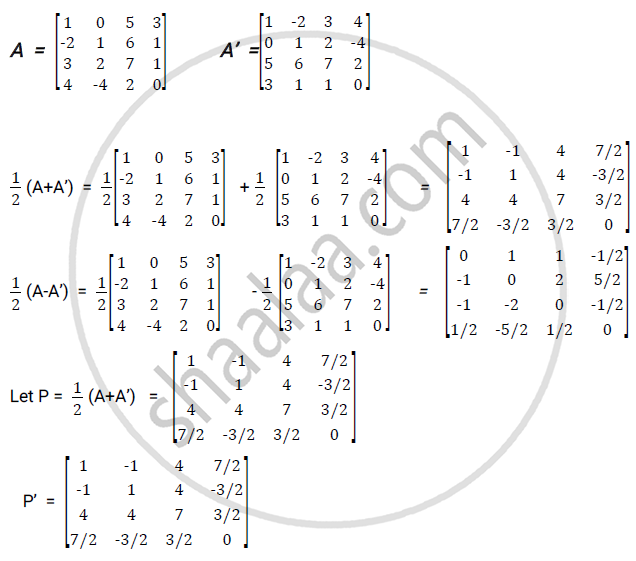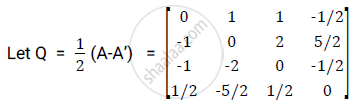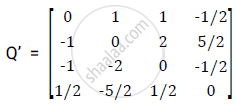# Express the Matrix as the Sum of Symmetric and Skew Symmetric Matrices. - Applied Mathematics 1

Sum

Express the matrix as the sum of symmetric and skew symmetric matrices.

#### SolutionHence P =P’.P is a symmetric matrix.Hence Q =Q’. Q is a skew symmetric matrix.